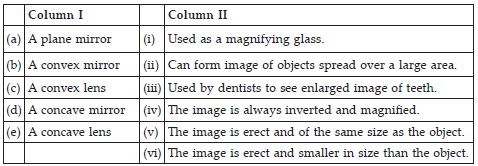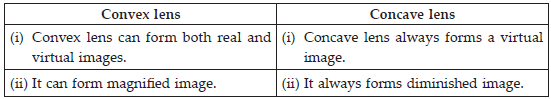Light (Physics) Class 7 - NCERT Questions
Q 1.

Fill in the blanks:
(A) An image that cannot be obtained on a screen is called _______.
(B) Image formed by a convex _______ is always virtual and smaller in size.
(C) An image formed by a ______ mirror is always of the same size as that of the object.
(D) An image which can be obtained on a screen is called a _______ image.
(E) An image formed by a concave _______ cannot be obtained on a screen.

SOLUTION:

(A) virtual image, (B) mirror, (C) plane, (D) real, (E) lens

Q 2.

Mark ′T′ if the statement is true and ′F′ if it is false:
(A) We can obtain an enlarged and erect image by a convex mirror. (T/F)
(B) A concave lens always form a virtual image. (T/F)
(C) We can obtain a real, enlarged and inverted image by a concave mirror. (T/F)
(D) A real image cannot be obtained on a screen. (T/F)
(E) A concave mirror always form a real image. (T/F)

SOLUTION:

(A) False, (B) True, (C) True, (D) False, (E) False

Q 3.

Match the items given in Column I with one or more items of Column II.SOLUTION:

(A)-(v); (B)-(ii), (vi); (C)-(i); (D)-(iii), (E)-(vi)

Q 4.

State the characteristics of the image formed by a plane mirror.

SOLUTION:

The image formed by a plane mirror has the following characteristics:
(i) The distance of the object in front of the mirror is same as the distance of the image behind the mirror.
(ii) The image formed by a plane mirror is virtual and erect.
(iii) The size of the image is equal to the object size.

Q 5.

Find out the letters of English alphabet or any other language known to you in which the image formed in a plane mirror appears exactly like the letter itself. Discuss your findings.

SOLUTION:

The letters of English alphabet which give exactly same image in a plane mirror like the letter itself are :
A, H, I, M, O, T, U, V, W, X, Y

Q 6.

What is a virtual image? Give one situation where a virtual image is formed.

SOLUTION:

The image formed by the plane mirror appears behind it. We cannot however touch it. Also, the image of the object cannot be obtained on a screen whether it is held in front of the mirror or behind it. Such types of images are not real. They are virtual images.

Q 7.

State two differences between a convex and a concave lens.

SOLUTION:Q 8.

Give one use each of a concave and a convex mirror.

SOLUTION:

Concave mirror : Used by dentists to obtain a large virtual image of the teeth.
Convex mirror : Used in vehicles as rear view mirrors, since they form the image of objects spread over a large area.

Q 9.

Which type of mirror can form a real image?

SOLUTION:

Concave mirror can form a real image.

Q 10.

Which type of lens forms always a virtual image?

SOLUTION:

Concave lens always forms a virtual image.

Q 11.

A virtual image larger than the object can be produced by a
(i) concave lens (ii) concave mirror (iii) convex mirror (iv) plane mirror

SOLUTION:

(ii)

Q 12.

David is observing his image in a plane mirror. The distance between the mirror and his image is 4 m. If he moves 1 m towards the mirror, then the distance between David and his image will be
(i) 3 m (ii) 5 m (iii) 6 m (iv) 8 m

SOLUTION:

(iii) : If David moves 1 m towards the mirror, then distance between his image and mirror = 4 – 1 = 3 m
So, distance between David and mirror = 3 m
Hence, distance between David and his image = 3 + 3 = 6 m

Q 13.

The rear view mirror of a car is a plane mirror. A driver is reversing his car at a speed of 2 m/s. The driver sees in his rear view mirror the image of a truck parked behind his car. The speed at which the image of the truck appears to approach the driver will be
(i) 1 m/s (ii) 2 m/s (iii) 4 m/s (iv) 8 m/s

SOLUTION:

(iii)